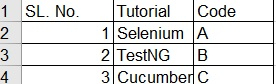# How to get all the values of a particular column based on a condition in a worksheet in Selenium with python?

We can get all the values of a particular column based on a condition in a worksheet in Selenium. Excel is a spreadsheet which is saved with the .xlsx extension. An excel workbook has multiple sheets and each sheet consists of rows and columns.

Out of all the worksheets, while we are accessing a particular sheet that is called as an active sheet. Each cell inside a sheet has a unique address which is a combination of row and column numbers.

The column number starts from alphabetic character A and row number starts from the number 1. A cell can contain numerous types of values and they are the main component of a worksheet.

To work with excel in Selenium with python, we need to take help of OpenPyXL library. This library is responsible for reading and writing operations on Excel, having the extensions like xlsx, xlsm, xltm, xltx.

To install OpenPyXL library, we have to execute the command pip install openpyxl. This is because OpenPyXL does not come by default with python. After this we should import openpyxl in our code and then we should be ready to interact with excel.

To get the values from a particular column, first of all we need to load the entire workbook by specifying the path where it is located. This is achieved with load_workbook() method. Next we need to identify the active sheet among all the worksheets with the help of active method.

Next we need to use the max_row method that gives the count of the number of occupied rows. Please note this method is to be used with the worksheet level object.

And we need to use the max_column method that gives the count of the number of occupied columns. Please note this method is to be used with the worksheet level object.

We need to iterate from 1 to the count of the maximum number of occupied rows to traverse through all the rows. Let us consider that our desired value is present in column 1. So we need to check if the value exists there.

If the value is present we need to iterate from 1 to the count of maximum number of occupied columns to traverse through all the columns.

Finally to retrieve all the values in that particular column, we need the help of row and column number and the cell method [which accepts the row and column number as arguments]. For example, to point to the cell corresponding to row 2 and column 3, we need to mention sheet.cell(row=2,column=3).

## Syntax

wrkbk = load_workbook("C:\work\SeleniumPython.xlsx")
# to identify the active sheet
sh = wrkbk.active
# identify the number of occupied rows
sh.max_row
# identify the number of occupied rows
sh.max_column

## Example

Coding Implementation to get all the values from a particular column.

import openpyxl
# load excel with its path
# to get the active work sheet
sh = wrkbk.active
# to print the maximum number of occupied rows in console
print(sh.max_row)
# to print the maximum number of occupied columns in console
print(sh.max_column)
# to get all the values from the excel and traverse through the rows
for r in range(1,max_row+1):
# to traverse through the columns
for c in range(i,max_column+1):
# to check the value in row 1
if(sh.cell(row=1, column=c).value == "Tutorial":
# to get all the values
print(sh.cell(row=r, column=c).value)

Excel data we are referring to −